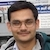# Angular Momentum of a ProjectileBy

The angular momentum of a projectile about the point of projection varies with time as \begin{align} L=\frac{1}{2} mu g \cos\theta\; t^2\nonumber \end{align} The magnitude of angular momentum at the highest point is \begin{align} L=\frac{mu^3\sin^2\theta\cos\theta}{2g}\nonumber \end{align}Projectile of Mass $m$ projected from the origin O.

## Angular Momentum of a Projectile at its Highest Point

The horizontal component of velocity at the highest point is $u\cos\theta$ and the vertical component is zero. The highest point is at a height \begin{align} H=\frac{u^2\sin^2\theta}{2g}\nonumber \end{align} The magnitude of angular momentum of the projectile about the origin at the highest point is \begin{align} L&=mr_\perp v=m H u\cos\theta\nonumber\\ &=\frac{mu^3\sin^2\theta\cos\theta}{2g}\nonumber \end{align}

## Angular Momentum of a Projectile at a Time $t$

The components of projectile's velocity at a time $t$ are \begin{align} v_x&=u\cos\theta\nonumber\\ v_y&=u\sin\theta-gt\nonumber \end{align} The components of projectile's displacement at a time $t$ are \begin{align} x&=u\cos\theta\;t\nonumber\\ y&=u\sin\theta\;t-\frac{1}{2}gt^2\nonumber \end{align} The angular momentum of the projectile about the origin at a time $t$ is \begin{align} \vec{L}&=m\vec{r}\times\vec{v}\nonumber\\ &=m(x\hat\imath+y\hat\jmath)\times(v_x\hat\imath+v_y\hat\jmath)\nonumber\\ &=-m(yv_x-xv_y)\hat{k}\nonumber\\ &=-\frac{1}{2} mug\cos\theta\;t^2\;\hat{k}\nonumber \end{align} The angular momentum points in $-\hat{k}$ direction. Its magnitude increases quadratically with time.

## Torque and Angular Momentum of a Projectile

The force on the projectile is $\vec{F}=-mg\hat{\jmath}$. The torque on the projectile about the origin is \begin{align} \vec{\tau}&=\vec{r}\times\vec{F}=-umg\cos\theta\;t \; \hat{k}\nonumber \end{align} The angular momentum is related to the torque by $\mathrm{d}\vec{L}=\vec{\tau}\mathrm{d}t$. Substitute $\vec{\tau}$ and integrate to get \begin{align} \vec{L}&=\int_0^t \vec{\tau}\;\mathrm{d}t\nonumber\\ &=-\int_0^t umg\cos\theta\; t \;\mathrm{d}t\;\hat{k}\nonumber\\ &=-\frac{1}{2} mug\cos\theta\;t^2\;\hat{k}\nonumber \end{align}

## Practice Problems

1. (IIT JEE 1990) A particle of mass $m$ is projected with a velocity $v$ making an angle of $45^\mathrm{o}$ with the horizontal. The magnitude of the angular momentum of the projectile about the point of projection when the particle is at its maximum height $h$ is
A. zero.
B. $\frac{mv^3}{4\sqrt{2} g}$
C. $\frac{mv^3}{\sqrt{2} g}$
D. $m\sqrt{2gh^3}$
2. The angular momentum of a projectile about the point of projection when it reaches the ground at the point Q is
A. $\frac{2mu^3\sin^2\theta\cos\theta}{g}$
B. $\frac{mu^3\sin^2\theta\cos\theta}{2g}$
C. $\frac{mu^3\sin\theta\cos^2\theta}{2g}$
D. zero